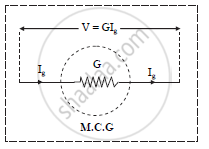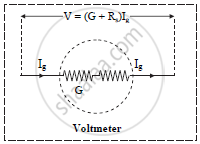HSC Science (Computer Science) 12th Board ExamMaharashtra State Board
Share

Explain How Moving Coil Galvanometer is Converted into a Voltmeter. Derive the Necessary Formula. - HSC Science (Computer Science) 12th Board Exam - Physics

Question

Explain how moving coil galvanometer is converted into a voltmeter. Derive the necessary formula.

Solution

a.   To use a M.C.G as a voltmeter, its resistance should be increased to a desired value and
an arrangement should be provided to measure large potential difference. This is
achieved by connecting a high resistance in series with the M.C.G.b. Let ‘G’ be the resistance of the galvanometer coil and ‘Ig’ be the maximum current
which can be passed through the galvanometer coil for full-scale deflection.

c. Let ‘V’ be the potential difference to be measured.
Let ‘Rs’ be the resistance connected in series with the galvanometer coil.

d. From Ohm’s law,
V = Ig (G + Rs)

G + Rs=V/I_g

Rs=V/I_g-G

Knowing V, Ig and G, value of Rs can be determined.

Is there an error in this question or solution?

APPEARS IN

2016-2017 (March) (with solutions)
Question 6.2 | 2.00 marks

Video TutorialsVIEW ALL 

Solution Explain How Moving Coil Galvanometer is Converted into a Voltmeter. Derive the Necessary Formula. Concept: Moving Coil Galvanometer.
S